# Mathematical constant

Mathematical constant

A mathematical constant is a special number, usually a real number, that is "significantly interesting in some way". Constants arise in many different areas of mathematics, with constants such as e and π occurring in such diverse contexts as geometry, number theory and calculus.

What it means for a constant to arise "naturally", and what makes a constant "interesting", is ultimately a matter of taste, and some mathematical constants are notable more for historical reasons than for their intrinsic mathematical interest. The more popular constants have been studied throughout the ages and computed to many decimal places.

All mathematical constants are definable numbers and usually are also computable numbers (Chaitin's constant being a significant exception).

## Common mathematical constants (some of which are also ubiquitous in science)

Ubiquitous in many different fields of science, such recurring constants include e, π and the Feigenbaum constants which are linked to the mathematical models used to describe physical phenomena, Euclidean geometry, analysis and logistic maps respectively. However, mathematical constants such as Apéry's constant and the Golden ratio occur unexpectedly outside of mathematics.

### Archimedes' constant πThe circumference of a circle with diameter 1 is π.

The constant π has a natural definition in Euclidean geometry (the ratio between the circumference and diameter of a circle), but may also be found in many different places in mathematics: for example the Gaussian integral in complex analysis, nth roots of unity in number theory and Cauchy distributions in probability. However, its universality is not limited to pure mathematics. Indeed, various formulae in physics, such as Heisenberg's uncertainty principle, and constants such as the cosmological constant include the constant π. The presence of π in physical principles, laws and formulae can have very simple explanations. For example, Coulomb's law, describing the inverse square proportionality of the magnitude of the electrostatic force between two electric charges and their distance, states that, in SI units,$F = \frac{1}{4\pi\varepsilon_0}\frac{\left|q_1 q_2\right|}{r^2}.$

### Euler's number e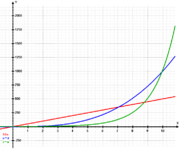Exponential growth (green) describes many physical phenomena.

Euler's Number e, also known as the exponential growth constant, appears in many areas of mathematics, and one possible definition of it is the value of the following expression:$e = \lim_{n\to\infty} \left( 1 + \frac{1}{n} \right)^n$

For example, the Swiss mathematician Jacob Bernoulli discovered that e arises in compound interest: An account that starts at \$1, and yields interest at annual rate R with continuous compounding, will accumulate to eR dollars at the end of one year. The constant e also has applications to probability theory, where it arises in a way not obviously related to exponential growth. Suppose that a gambler plays a slot machine with a one in n probability of winning, and plays it n times. Then, for large n (such as a million) the probability that the gambler will win nothing at all is (approximately) 1/e.

Another application of e, discovered in part by Jacob Bernoulli along with French mathematician Pierre Raymond de Montmort, is in the problem of derangements, also known as the hat check problem. Here n guests are invited to a party, and at the door each guest checks his hat with the butler who then places them into labelled boxes. But the butler does not know the name of the guests, and so must put them into boxes selected at random. The problem of de Montmort is: what is the probability that none of the hats gets put into the right box. The answer is$p_n = 1-\frac{1}{1!}+\frac{1}{2!}-\frac{1}{3!}+\cdots+(-1)^n\frac{1}{n!}$

and as n tends to infinity, pn approaches 1/e.

### The Feigenbaum constants α and δ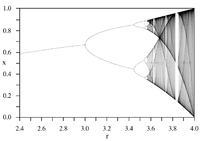Bifurcation diagram of the logistic map.

Iterations of continuous maps serve as the simplest examples of models for dynamical systems. Named after mathematical physicist Mitchell Feigenbaum, the two Feigenbaum constants appear in such iterative processes: they are mathematical invariants of logistic maps with quadratic maximum points and their bifurcation diagrams.

The logistic map is a polynomial mapping, often cited as an archetypal example of how chaotic behaviour can arise from very simple non-linear dynamical equations. The map was popularized in a seminal 1976 paper by the English biologist Robert May, in part as a discrete-time demographic model analogous to the logistic equation first created by Pierre François Verhulst. The difference equation is intended to capture the two effects of reproduction and starvation.

### Apéry's constant ζ(3)$\zeta(3) = 1 + \frac{1}{2^3} + \frac{1}{3^3} + \frac{1}{4^3} + \dots$

Despite being a special value of the Riemann zeta function, Apéry's constant arises naturally in a number of physical problems, including in the second- and third-order terms of the electron's gyromagnetic ratio, computed using quantum electrodynamics. Also, the neuroscientist David Eagleman noted that$\sqrt{m_n/m_e}\approxeq\frac{3}{\sqrt{\varphi}-\zeta(3)},$

where mn,me are the neutron mass, the electron mass and the Golden ratio respectively.

### The golden ratio φ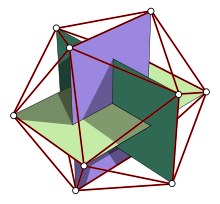Golden rectangles in an icosahedron$F\left(n\right)=\frac{\varphi^n-(1-\varphi)^n}{\sqrt 5}$
An explicit formula for the nth Fibonacci number involving the golden ratio.

The number φ turns up frequently in geometry, particularly in figures with pentagonal symmetry. Indeed, the length of a regular pentagon's diagonal is φ times its side. The vertices of a regular icosahedron are those of three mutually orthogonal golden rectangles. Also, it appears in the Fibonacci sequence, related to growth by recursion. It is approximately equal to 1.61803398874, or, more precisely$\scriptstyle\frac{1+\sqrt{5}}{2}.$

Adolf Zeising, whose main interests were mathematics and philosophy, found the golden ratio expressed in the arrangement of branches along the stems of plants and of veins in leaves. He extended his research to the skeletons of animals and the branchings of their veins and nerves, to the proportions of chemical compounds and the geometry of crystals, even to the use of proportion in artistic endeavours. In these phenomena he saw the golden ratio operating as a universal law. Zeising wrote in 1854:

[The Golden Ratio is a universal law] in which is contained the ground-principle of all formative striving for beauty and completeness in the realms of both nature and art, and which permeates, as a paramount spiritual ideal, all structures, forms and proportions, whether cosmic or individual, organic or inorganic, acoustic or optical; which finds its fullest realization, however, in the human form.

### The Euler–Mascheroni constant γ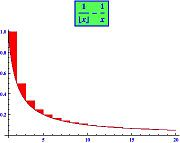The area between the two curves (red) tends to a limit.

The Euler–Mascheroni constant is a recurring constant in number theory. The French mathematician Charles Jean de la Vallée-Poussin proved in 1898 that when taking any positive integer n and dividing it by each positive integer m less than n, the average fraction by which the quotient n/m falls short of the next integer tends to γ as n tends to infinity. Surprisingly, this average doesn't tend to one half. The Euler–Mascheroni constant also appears in Merten's third theorem and has relations to the gamma function, the zeta function and many different integrals and series. The definition of the Euler–Mascheroni constant exhibits a close link between the discrete and the continuous (see curves on the left).

### Conway's constant λ$\begin{matrix} 1 \\ 11 \\ 21 \\ 1211 \\ 111221 \\ 312211 \\ \vdots \end{matrix}$

Conway's constant is the invariant growth rate of all derived strings similar to the look-and-say sequence (except two trivial ones). It is given by the unique positive real root of a polynomial of degree 71 with integer coefficients.

### Khinchin's constant K

If a real number r is written as a simple continued fraction:$r=a_0+\dfrac{1}{a_1+\dfrac{1}{a_2+\dfrac{1}{a_3+\cdots}}},$

where ak are natural numbers for all k

then, as the Russian mathematician Aleksandr Khinchin proved in 1934, the limit as n tends to infinity of the geometric mean: (a1a2...an)1/n exists and is a constant, Khinchin's constant, except for a set of measure 0.

## Mathematical curiosities and unspecified constants

### Simple representatives of sets of numbers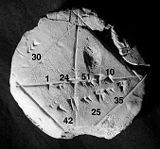This Babylonian clay tablet gives an approximation of the square root of 2 in four sexagesimal figures, which is about six decimal figures.$c=\sum_{j=1}^\infty 10^{-j!}=0.\underbrace{\overbrace{110001}^{3!\text{ digits}}000000000000000001}_{4!\text{ digits}}000\dots\,$
Liouville's constant is a simple example of a transcendental number.

Some constants, such as the square root of 2, Liouville's constant and Champernowne constant:$C_{10} = \color{black}0.\color{blue}1\color{black}2\color{blue}3\color{black}4\color{blue}5\color{black}6\color{blue}7\color{black}8\color{blue}9\color{black}10\color{blue}11\color{black}12\color{blue}13\color{black}14\color{blue}15\color{black}16\dots$

are not important mathematical invariants but retain interest being simple representatives of special sets of numbers, the irrational numbers, the transcendental numbers and the normal numbers (in base 10) respectively. The discovery of the irrational numbers is usually attributed to the Pythagorean Hippasus of Metapontum who proved, most likely geometrically, the irrationality of the square root of 2. As for Liouville's constant, named after French mathematician Joseph Liouville, it was the first number to be proven transcendental.

### Chaitin's constant Ω

In the computer science subfield of algorithmic information theory, Chaitin's constant is the real number representing the probability that a randomly chosen Turing machine will halt, formed from a construction due to Argentine-American mathematician and computer scientist Gregory Chaitin. Chaitin's constant, though not being computable, has been proven to be transcendental and normal. Chaitin's constant is not universal, depending heavily on the numerical encoding used for Turing machines; however, its interesting properties are independent of the encoding.

### Unspecified constants

When unspecified, constants indicate classes of similar objects, commonly functions, all equal up to a constant—technically speaking, this is may be viewed as 'similarity up to a constant'. Such constants appear frequently when dealing with integrals and differential equations. Though unspecified, they have a specific value, which often isn't important.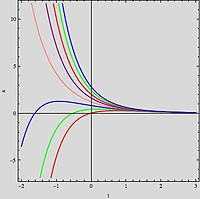Solutions with different constants of integration of$y'(x)=-2y+e^{-x}\,$.

#### In integrals

Indefinite integrals are called indefinite because their solutions are only unique up to a constant. For example, when working over the field of real numbers$\int\cos x\ dx=\sin x+C$

where C, the constant of integration, is an arbitrary fixed real number. In other words, whatever the value of C, differentiating sin x + C with respect to x always yields cos x.

#### In differential equations

In a similar fashion, constants appear in the solutions to differential equations where not enough initial values or boundary conditions are given. For example, the ordinary differential equation y' = y(x) has solution Cex where C is an arbitrary constant.

When dealing with partial differential equations, the constants may be functions, constant with respect to some variables (but not necessarily all of them). For example, the PDE$\frac{\partial f(x,y)}{\partial x}=0$

has solutions f(x,y)=C(y), where C(y) is an arbitrary function in the variable y.

## Notation

### Representing constants

It is common to express the numerical value of a constant by giving its decimal representation (or just the first few digits of it). For two reasons this representation may cause problems. First, even though rational numbers all have a finite or ever-repeating decimal expansion, irrational numbers don't have such an expression making them impossible to completely describe in this manner. Also, the decimal expansion of a number is not necessarily unique. For example, the two representations 0.999... and 1 are equivalent in the sense that they represent the same number.

Calculating digits of the decimal expansion of constants has been a common enterprise for many centuries. For example, German mathematician Ludolph van Ceulen of the 16th century spent a major part of his life calculating the first 35 digits of pi. Using computers and supercomputers, some of the mathematical constants, including π, e, and the square root of 2, have been computed to more than one hundred billion digits. Fast algorithms have been developed, some of which — as for Apéry's constant — are unexpectedly fast.$G=\left . \begin{matrix} 3 \underbrace{ \uparrow \ldots \uparrow } 3 \\ \underbrace{\vdots } \\ 3 \uparrow\uparrow\uparrow\uparrow 3 \end{matrix} \right \} \text{64 layers}$

Some constants differ so much from the usual kind that a new notation has been invented to represent them reasonably. Graham's number illustrates this as Knuth's up-arrow notation is used.

It may be of interest to represent them using continued fractions to perform various studies, including statistical analysis. Many mathematical constants have an analytic form, that is they can constructed using well-known operations that lend themselves readily to calculation. Not all constants have known analytic forms, though; Grossman's constant and Foias' constant are examples.

### Symbolizing and naming of constants

Symbolizing constants with letters is a frequent means of making the notation more concise. A standard convention, instigated by Leonhard Euler in the 18th century, is to use lower case letters from the beginning of the Latin alphabet$a,b,c,\dots\,$ or the Greek alphabet$\alpha,\beta,\,\gamma,\dots\,$ when dealing with constants in general.

Erdős–Borwein constant$E_B\,$
Embree-Trefethen constant$\beta*\,$
Brun's constant for twin prime$B_2\,$
Champernowne constants Cb
cardinal number aleph naught$\aleph_0$
Examples of different kinds of notation for constants.

However, for more important constants, the symbols may be more complex and have an extra letter, an asterisk, a number, a lemniscate or use different alphabets such as Hebrew, Cyrillic or Gothic.$\mathrm{googol}=10^{100}\,\ ,\ \mathrm{googolplex}=10^\mathrm{googol}=10^{10^{100}}\,$

Sometimes, the symbol representing a constant is a whole word. For example, American mathematician Edward Kasner's 9-year-old nephew coined the names googol and googolplex.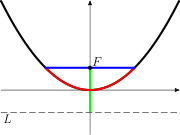The universal parabolic constant is the ratio, for any parabola, of the arc length of the parabolic segment (red) formed by the latus rectum (blue) to the focal parameter (green).

The names are either related to the meaning of the constant (universal parabolic constant, twin prime constant, ...) or to a specific person (Sierpiński's constant, Josephson constant, ...).

## Table of selected mathematical constants

Abbreviations used:

R – Rational number, I – Irrational number (may be algebraic or transcendental), A – Algebraic number (irrational), T - Transcendental number (irrational)
Gen – General, NuT – Number theory, ChT – Chaos theory, Com – Combinatorics, Inf – Information theory, Ana – Mathematical analysis
Symbol Value Name Field N First Described # of Known Digits
0
= 0 Zero Gen R c. 7th–5th century BCE N/A
1
= 1 One, Unity Gen R N/A
i
=$\sqrt{-1}$ Imaginary unit Gen, Ana A 16th century N/A
π
≈ 3.14159 26535 89793 23846 26433 83279 50288 Pi, Archimedes' constant or Ludolph's number Gen, Ana T by c. 2000 BCE 10,000,000,000,000
e
≈ 2.71828 18284 59045 23536 02874 71352 66249 Napier's constant, or Euler's number, base of Natural logarithm Gen, Ana T 1618 100,000,000,000
2
≈ 1.41421 35623 73095 04880 16887 24209 69807 Pythagoras' constant, square root of 2 Gen A by c. 800 BCE 137,438,953,444
3
≈ 1.73205 08075 68877 29352 74463 41505 87236 Theodorus' constant, square root of 3 Gen A by c. 800 BCE
5
≈ 2.23606 79774 99789 69640 91736 68731 27623 square root of 5 Gen A by c. 800 BCE 1,000,000
γ
≈ 0.57721 56649 01532 86060 65120 90082 40243 Euler–Mascheroni constant Gen, NuT 1735 14,922,244,771
ϕ
≈ 1.61803 39887 49894 84820 45868 34365 63811 Golden ratio Gen A by 3rd century BCE 100,000,000,000
ρ
≈ 1.32471 79572 44746 02596 09088 54478 09734 Plastic constant NuT A 1928
β*
≈ 0.70258 Embree–Trefethen constant NuT
δ
≈ 4.66920 16091 02990 67185 32038 20466 20161 Feigenbaum constant ChT 1975
α
≈ 2.50290 78750 95892 82228 39028 73218 21578 Feigenbaum constant ChT
C2
≈ 0.66016 18158 46869 57392 78121 10014 55577 Twin prime constant NuT 5,020
M1
≈ 0.26149 72128 47642 78375 54268 38608 69585 Meissel–Mertens constant NuT 1866
1874
8,010
B2
≈ 1.90216 05823 Brun's constant for twin primes NuT 1919 10
B4
≈ 0.87058 83800 Brun's constant for prime quadruplets NuT
Λ
≥ –2.7 • 10−9 de Bruijn–Newman constant NuT 1950? none
K
≈ 0.91596 55941 77219 01505 46035 14932 38411 Catalan's constant Com 15,510,000,000
K
≈ 0.76422 36535 89220 66299 06987 31250 09232 Landau–Ramanujan constant NuT 30,010
K
≈ 1.13198 824 Viswanath's constant NuT 8
L
= 1 Legendre's constant NuT R N/A
μ
≈ 1.45136 92348 83381 05028 39684 85892 02744 Ramanujan–Soldner constant NuT 75,500
EB
≈ 1.60669 51524 15291 76378 33015 23190 92458 Erdős–Borwein constant NuT I
β
≈ 0.28016 94990 23869 13303 Bernstein's constant Ana
λ
≈ 0.30366 30028 98732 65859 74481 21901 55623 Gauss–Kuzmin–Wirsing constant Com 1974 385
σ
≈ 0.35323 63718 54995 98454 Hafner–Sarnak–McCurley constant NuT 1993
λ, μ
≈ 0.62432 99885 43550 87099 29363 83100 83724 Golomb–Dickman constant Com, NuT 1930
1964
≈ 0.64341 05463 Cahen's constant T 1891 4000
≈ 0.66274 34193 49181 58097 47420 97109 25290 Laplace limit
Λ
≈ 1.09868 58055 Lengyel's constant Com 1992
≈ 3.27582 29187 21811 15978 76818 82453 84386 Lévy's constant NuT
ζ(3)
≈ 1.20205 69031 59594 28539 97381 61511 44999 Apéry's constant I 1979 15,510,000,000
θ
≈ 1.30637 78838 63080 69046 86144 92602 60571 Mills' constant NuT 1947 6850
≈ 1.45607 49485 82689 67139 95953 51116 54356 Backhouse's constant
≈ 1.46707 80794 Porter's constant NuT 1975
≈ 1.53960 07178 Lieb's square ice constant Com 1967
≈ 1.70521 11401 05367 76428 85514 53434 50816 Niven's constant NuT 1969
K
≈ 2.58498 17595 79253 21706 58935 87383 17116 Sierpiński's constant
≈ 2.68545 20010 65306 44530 97148 35481 79569 Khinchin's constant NuT 1934 7350
F
≈ 2.80777 02420 28519 36522 15011 86557 77293 Fransén-Robinson constant Ana
L
≈ 0.5 Landau's constant Ana 1
P2
≈ 2.29558 71493 92638 07403 42980 49189 49039 Universal parabolic constant Gen T
Ω
≈ 0.56714 32904 09783 87299 99686 62210 35555 Omega constant Ana T
MRB
≈ 0.187859 MRB constant NuT 3:13GMT 1/11/1999 300,000 

Wikimedia Foundation. 2010.

### Look at other dictionaries:

• e (mathematical constant) — Euler s number redirects here. For γ, a constant in number theory, see Euler s constant. For other uses, see List of topics named after Leonhard Euler#Euler numbers. e is the unique number a, such that the derivative of the exponential function… …   Wikipedia

• E (mathematical constant) — The mathematical constant e is the unique real number such that the function e x has the same value as the slope of the tangent line, for all values of x . [Keisler, H.J. [http://www.vias.org/calculus/08 exp log functions 03 01.html Derivatives… …   Wikipedia

• Constant — or The Constant may refer to: Contents 1 In Mathematics 2 Other concepts 3 People 4 Organization 5 …   Wikipedia

• Constant (disambiguation) — A constant is something that does not change, over time or otherwise: a fixed value. In most fields of discourse the term is an antonym of variable , but in mathematical parlance a mathematical variable may sometimes also be called a constant.… …   Wikipedia

• Mathematical constants (sorted by continued fraction representation) — This is a list of mathematical constants sorted by their representations as continued fractions:(Constants known to be irrational have infinite continued fractions: their last term is ....)Symbol NumeralNumber setValue Continued fraction… …   Wikipedia

• Constant (mathematics) — In mathematics, a constant is a non varying value, i.e. completely fixed or fixed in the context of use. The term usually occurs in opposition to variable (i.e. variable quantity), which is a symbol that stands for a value that may vary. In… …   Wikipedia

• Mathematical principles of reinforcement — (MPR) are a set of mathematical equations that attempt to describe and predict the most fundamental aspects of behavior. The three key principles of MPR, arousal, constraint, and coupling, describe how incentives motivate responding, how time… …   Wikipedia

• Mathematical finance — is a field of applied mathematics, concerned with financial markets. The subject has a close relationship with the discipline of financial economics, which is concerned with much of the underlying theory. Generally, mathematical finance will… …   Wikipedia

• Constant — Con stant, n. 1. That which is not subject to change; that which is invariable. [1913 Webster] 2. (Math.) A quantity that does not change its value; used in countradistinction to {variable}. [1913 Webster] 3. (Astron.) A number whose value, when… …   The Collaborative International Dictionary of English

• Constant of aberration — Constant Con stant, n. 1. That which is not subject to change; that which is invariable. [1913 Webster] 2. (Math.) A quantity that does not change its value; used in countradistinction to {variable}. [1913 Webster] 3. (Astron.) A number whose… …   The Collaborative International Dictionary of English